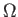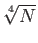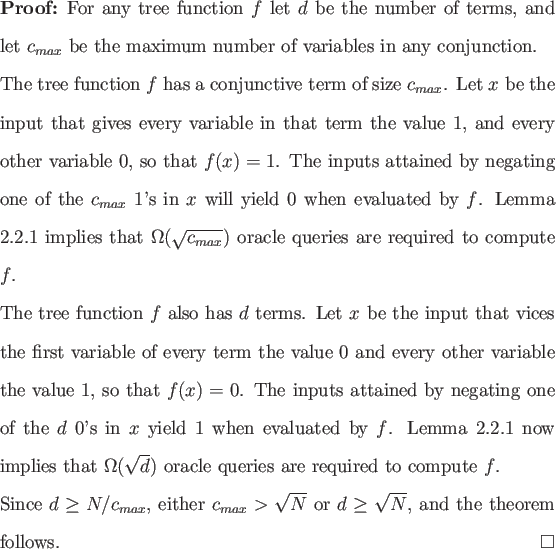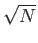Next: Nondeterministic Decision Tree Complexity Up: Boolean Functions Previous: Boolean Functions   Contents

# Tree Functions

Tree functions are Boolean functions of N bits that can be written as a disjunction of conjunctions in which each of the N variables occurs exactly once. Tree functions are evasive in the classical case .

Theorem 4.1.1() oracle queries are required to compute any tree function of N bits in the bounded error setting.This lower bound is a special case of the() lower bound for monotone functions proved by Beals et al. , as tree functions are monotone functions with decision tree complexity N. Here we see for the first time a gap that is not quadratic with the classical case. While this lower bound agrees with the result for monotone functions, the author believes it is not asymptotically tight. Whether there is an() lower bound for computing N-bit tree functions is an open question.Next: Nondeterministic Decision Tree Complexity Up: Boolean Functions Previous: Boolean Functions   Contents
Matthew Hayward Lower Query Bounds in the Quantum Oracle Model GitHub Repository